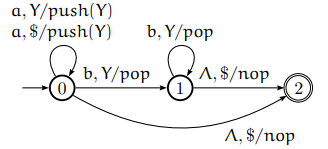# What happens when a String is accepted or rejected by NPDA?

Data Structure AlgorithmsComputer ScienceComputers

A string is accepted by an Non-deterministic Push down Automata (NPDA), if there is some path (i.e., sequence of instructions) from the start state to a final state that consumes all the letters of the string. Otherwise, the string is rejected by the NPDA.

The language of an NPDA is the set of all strings that it accepts.

An input string rejected by the NPDA under following conditions −

• If reading an input string finishes without reaching a final state.

• If for a current state/symbol on the stack/input symbol there is no transition.

• If it attempts to pop the empty stack.

Example

Build an NPDA which recognises the context-free language

L = {anbn| n > 0}.

Steps

Follow the steps given below to build a NPDA −

• Begin reading the string, and for each a read, push a Y onto the stack.

• On the first b change states, and begin removing one Y from the stack for each b.

• If you reach the end of the input and have just cleared the stack, accept the string.

• Otherwise, reject. For example, if the stack runs out before the input –more b’s than a’s.

Push down automata for { anbn , n>=0}

Three states − 0 (start), 1, 2(final)

Input alphabet − {a,b}

Stack alphabet − { Y,$} The push down automata will be as follows −There are six allowed instructions for this NPDA, which are as follows − • T1 − (0, a,$, push(Y), 0)

• T2 − (0, a, Y, push(Y), 0)

• T3 − (0, ∧, $, nop, 2) • T4 − (0, b, Y, pop, 1) • T5 − (1, b, Y, pop, 1) • T6 − (1, ∧,$, nop, 2)

Reading \$ corresponds to checking if the stack is empty.